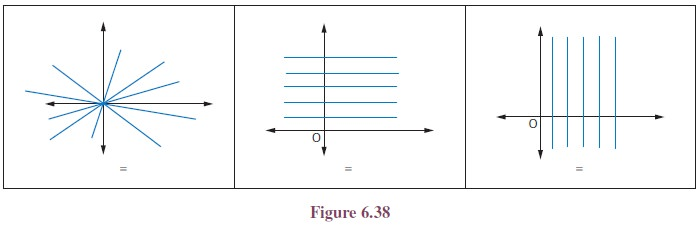Home | | Maths 11th std | Angle between two straight lines

# Angle between two straight lines

Two straight lines in a plane would either be parallel or coincide or intersect. Normally when two straight lines intersect, they form two angles at the point of intersection.

Angle between two straight lines

Two straight lines in a plane would either be parallel or coincide or intersect. Normally when two straight lines intersect, they form two angles at the point of intersection. One is an acute angle and another is an obtuse angle or equal. Both these angles would be supplements(Sum equals 180) of each other. By definition, when we say ‘angle between two straight lines’ we mean the acute angle between the two lines.be the equations of two straight lines and let these two lines make angles θ1and θ2 with x- axis.## 1. Condition for Parallel Lines## 2. Condition for perpendicular Lines## 3. Position of a point with respect to a straight line

Any line ax + by + c = 0 (c = 0),divides the whole plane in to two parts:

(i) one containing the origin called origin side of the line and

(ii) the other not containing the origin called non-origin side of the line.

A point P (x1, y1) is on the origin side or non-origin side of the line ax + by + c = 0 (c ¹ 0), according as ax1 + by1 + c and c are of the same sign or opposite sign.

If c > 0, then P (x1, y1) is on the origin side or non orign side of the line ax + by + c = 0 , according as ax1 + by1 + c is positive or negative.## 4. Distance Formulas

Let us develop formulas to find the distance between

i.            two points

ii.            a point to a line

iii.            two parallel linesThis result shows from the fact that the angle in a semi circle is a right angle.

## 5. Family of lines

All lines follow a specific condition are called a family of lines. The following example shows some families of straight lines.(where m.h, and k are arbitrary constants).It may seem that the equation of a straight line ax + by + c = 0 contains three arbitrary constants. In fact, it is not so. On dividing it by b (or a, whichever is non-zero), we getThe above equation can be written as slope and intercept form.

It follows that the equation of a straight line contains two arbitrary constants, and the number of these arbitrary constants cannot be decreased further. Thus, the equation of every straight line contains two arbitrary constants; consequently, two conditions are needed to determine the equation of a straight line uniquely.

One condition yields a linear relation among two arbitrary constants and hence each arbitrary constant determines the other. Therefore, the lines which satisfy one condition contain a single arbitrary constant. Such a system of lines is called one parameter family of lines and the unknown arbitrary constant is called, the parameter.

Let us now discuss the three types of families of straight lines, using y = mx + b. First two types are one parameter families and third one is two parameters families

·        when m is arbitrary and b is a fixed constant.

·        when b is arbitrary and m is a fixed constant.

·        when both m and b are arbitrary

## 6. One parameter families

(i) when m is arbitrary and b is a fixed constantLet us find the family of equations of straight lines for the line y = m x + b by considering m is arbitrary constant and b is a fixed constant say b = 5. Therefore the equation, for different real values of m, represents a family of lines with y-intercept 5 units. A few members of this family are shown in figure. For example, in this diagram the slope m takes -1, -2, 1/3, 1 and 4.(ii) when b is arbitrary and m is a fixed constant.

As discussed above, suppose b is arbitrary constant and m is a fixed constant say m = 2,the equation y = m x + b becomes = 2x + b. For different real values of b, a family of lines can be obtained with slope 2. A few members of this family are shown in the figure. For example, in this diagram b can take values 3, −1, 0, 1, 2, 3 and 4.Two special cases family of parallel lines and family of perpendicular lines are given below## 7. Two parameters familiesTags : Definition, Equation Formula, Solved Example Problems, Exercise | Analytical Geometry | Mathematics , 11th Mathematics : UNIT 6 : Two Dimensional Analytical Geometry
Study Material, Lecturing Notes, Assignment, Reference, Wiki description explanation, brief detail
11th Mathematics : UNIT 6 : Two Dimensional Analytical Geometry : Angle between two straight lines | Definition, Equation Formula, Solved Example Problems, Exercise | Analytical Geometry | Mathematics